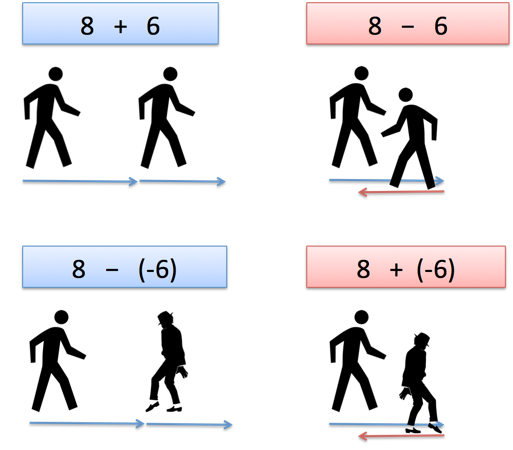Q&A

# How can I intuit $\dfrac{a - b}{c - d} \equiv \dfrac{{\color{red}{-}}(b - a)}{{\color{red}{-}}(d - c)} \equiv \dfrac{b - a}{d - c}$?

+1
−2

I'm not asking about algebra here which I can effortlessly effectuate. If helpful, let's intuit subtraction as facing backward, and the negative sign as backward steps. How does this intuition assist us to intuit $\dfrac{a - b}{c - d} \equiv \dfrac{{\color{red}{-}}(b - a)}{{\color{red}{-}}(d - c)} \equiv \dfrac{b - a}{d - c}$?

Ah! The addition/subtraction tells us which way to face, and the positive/negative tells us if our steps will be forward or backward (regardless of the way we're facing).In a sense, the addition/subtraction acts as a verb ("face forward" or "face backward"), and the positive/negative acts as an adjective ("regular steps" or "backwards steps"). Or maybe it's an adverb, modifying how we walk (walk forwardly, walk backwardly). You get the idea.

Why does this post require moderator attention?
Why should this post be closed?

+0
−0

(1)

$$\dfrac{a - b}{c - d}$$As the image depicts, $(a - b)$ means $a$ steps forward and $b$ steps backward which results in net $(a - b)$ steps, and dividing it by $(c - d)$ gives the number of steps (of length $(c - d)$) in $(a - b)$. Intuitive Meaning of Division can be found here.

(2) $$\dfrac{{{-}}(b - a)}{{{-}}(d - c)}$$$-(b - a) \implies -[-(a-b)] \implies -(-a)+(-b)$ which means $a$ steps forward by facing backward and similarly $b$ steps backward which again results in net $(a - b)$ steps, dividing it by $-[-(c - d)]$ (here the negative sign indicates the way length $(c - d)$ was measured. See the figure) gives the number of steps (of length $(c - d)$) in $(a - b)$. Just like (1)

(3) $$\dfrac{b - a}{d - c}$$Now can you intuite how is $\dfrac{b - a}{d - c}$ related to $\dfrac{a - b}{c - d}$ ?

P.S.: I have used 'length' in place of 'steps' in some sentences, this was done to not make the explanation complex. They don't really mean the same but are closely related.

Why does this post require moderator attention?